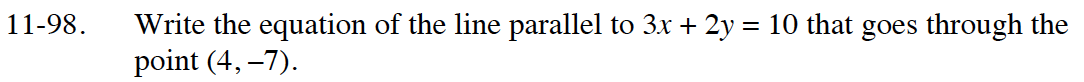Home > CC4 > Chapter 11 > Lesson 11.2.4 > Problem11-98

11-98.

Write the equation of the line parallel to 3x + 2y = 10 that goes through the point (4, −7). 11-98 HW eTool (Desmos). Homework Help ✎Change the equation of the given line to 'y = mx + b' form and identify the slope.
Then use the given point to find the equation of the line.

Use the eTool below to solve the problem.
Click the link at the right to view full version of the eTool: Int1 11-98 HW eTool.